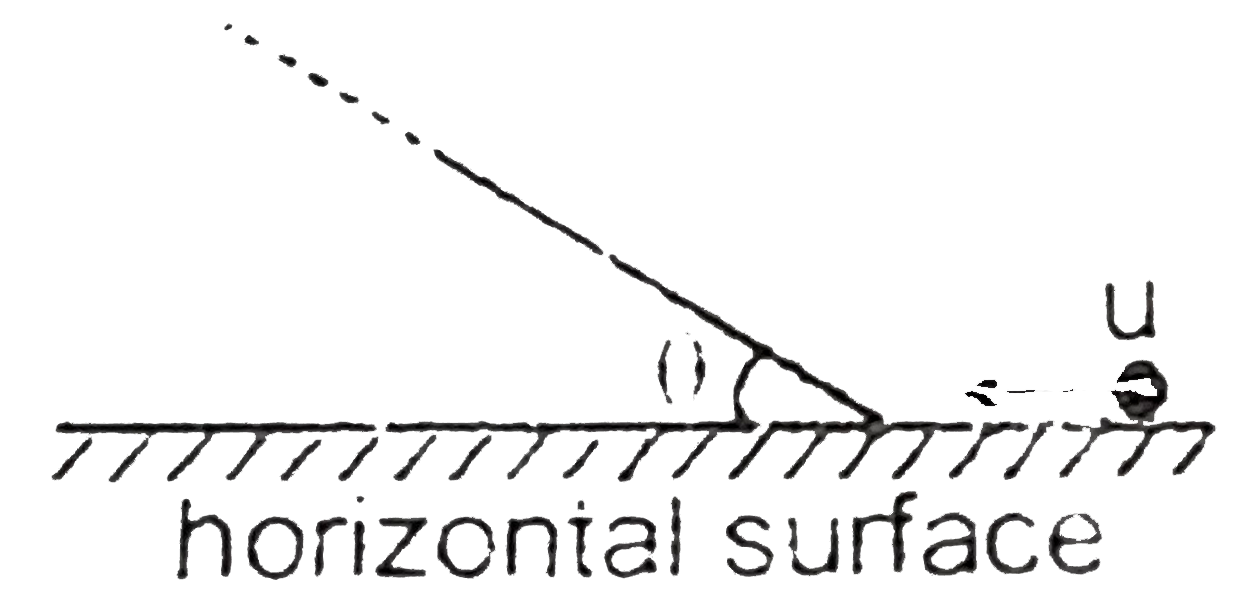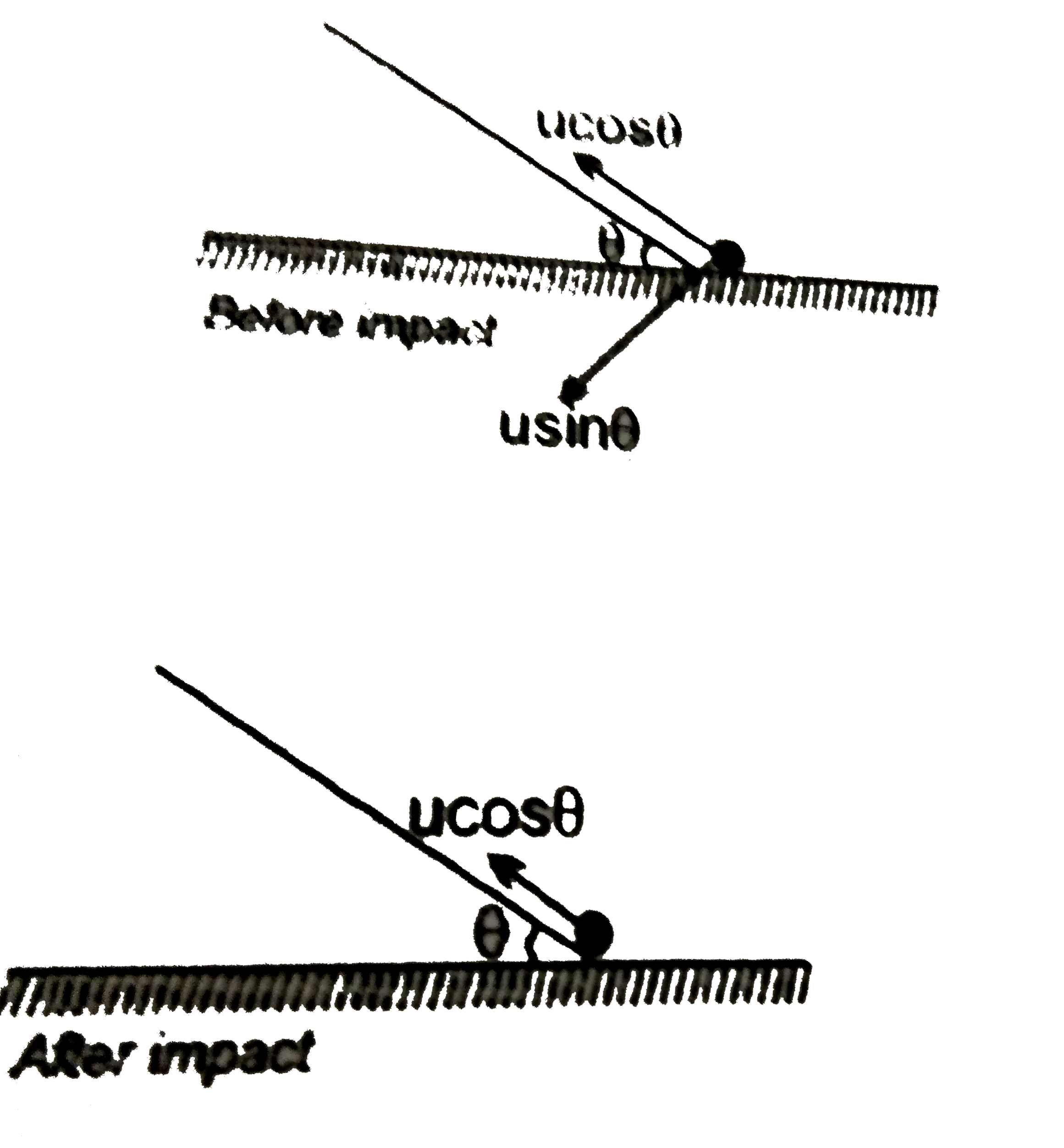Question

Updated on:30/05/2023

# A particle of mass m is given initial horizontal velocity of magnitude u as shown in the figure. It transfers to the fixed inclined plane without a jump, that is, its trajectory changes sharply from the horizontal line to the inclined line. All the surfaces are smooth and90∘≥θ>0∘. Then the height to which the particle shall rise on the inclined plane (assume the length of the inclined plane to be very large)Text Solution

A
increases with increase in θ
B
decreases with increase in θ
C
is independent of θ
D
data insufficient
Solution
Just before the particle transfers to inclined surface, we resolve its velocity along and normal to the plane.For the trajectory of the particles to sharply change from the horizontal line to be inclined line, the impact of the particle with inclined plane should reduce the usinθcomponent of velocity to zero. Hence the particle moves up the incline with speed ucosθ.
Hence as θ increases, the height to which the particle rises shall decrease.

Transcript

hello everyone so the question is a particle of mass M is given initial horizontal velocity of magnitude you as shown in the figure are you can see that this is the particle of mass M the mass of This particle is am and the initial velocity is given as you can see in the figure it transfers to the fix incline plane without observe that is its trajectory changes sharply from the horizontal line to an inclined plane all the surfaces are smooth and 90 degree is greater than theta greater than zero degree the angle of theta is greater than or equal to 90 and greater than zero then the height to which the particle Shall Rise on the inclined plane as you that the length of the incline planned to be very large we assume that the length of this plane is very low hai and we have to find out the height to which the particle Shall Rise on the inclined plane with these angles ok so there are some options
in the question and we have to tell that either the height is increases with increase in theatre or hide decreases with increasing theatre or independent of theatre or data in sufficient for education the diagram this is a particle of mass M which is moving initially moving with a velocity of you at this point when its path is changing in projectile motion if I take this is tangent then there is two components of this velocity first component is because its angle is theta this component is you cos theta and this component is you sin theta at this point the velocity of the particle is having two components first component is cos theta and second component is used in theatre now you can see that you cos theta is less than you because if the value of theta is increase that
is cos theta is decrease when cos theta is decrees that means velocity also decrease that means if I increase the value of theta that means cos theta will decrease and velocity is also decrease when velocity is decreased that means This particle is reaches less light reaches less height so I can say that with increasing theatre height is decrease or increase with increasing in so second option is correct now there is a second method also as you can see that height is given by the formula as equals to UT plus half a t square you all know that height is given by UT plus half sorry it's half a t square this is the formula of height of distance travelled by the particle now you can see that sorry half a
square now the gravitation the acceleration is which is acting on this particle is basically gravity acceleration due to gravity and that is acting down this is ji when this is ji that means there is a component of which is sin theta in this direction the component of gravity acceleration working as sin theta so I can write distance as equals to velocity is you cos theta time - because this is acting downwards what is minus half value of sin theta of 80 square so it is square now you can see if I increase the value of theta in case if I increase the value of theta that means cos theta will decrease that mean
this whole value is decrease similarly when I increase value of theta sin theta is when I increase value of theta then sin theta is increase but this value is in negative that means the net value or over L value is decrease overall value if I see that overall value is decrease education the in this equation if I increase theta then this value because cos theta is decrease at that time dismiss this is decreased this whole value decrease 2 cos theta it but this value half ji Centre because when I increase theta sin theta is increased for this value is negative when this value is in negative that means the overall value is comes as negative that means when we increase theta the height is basically decrease so I can write the second option is correct that height is decreases with increase in theatre ok I hope you all
standard thank you